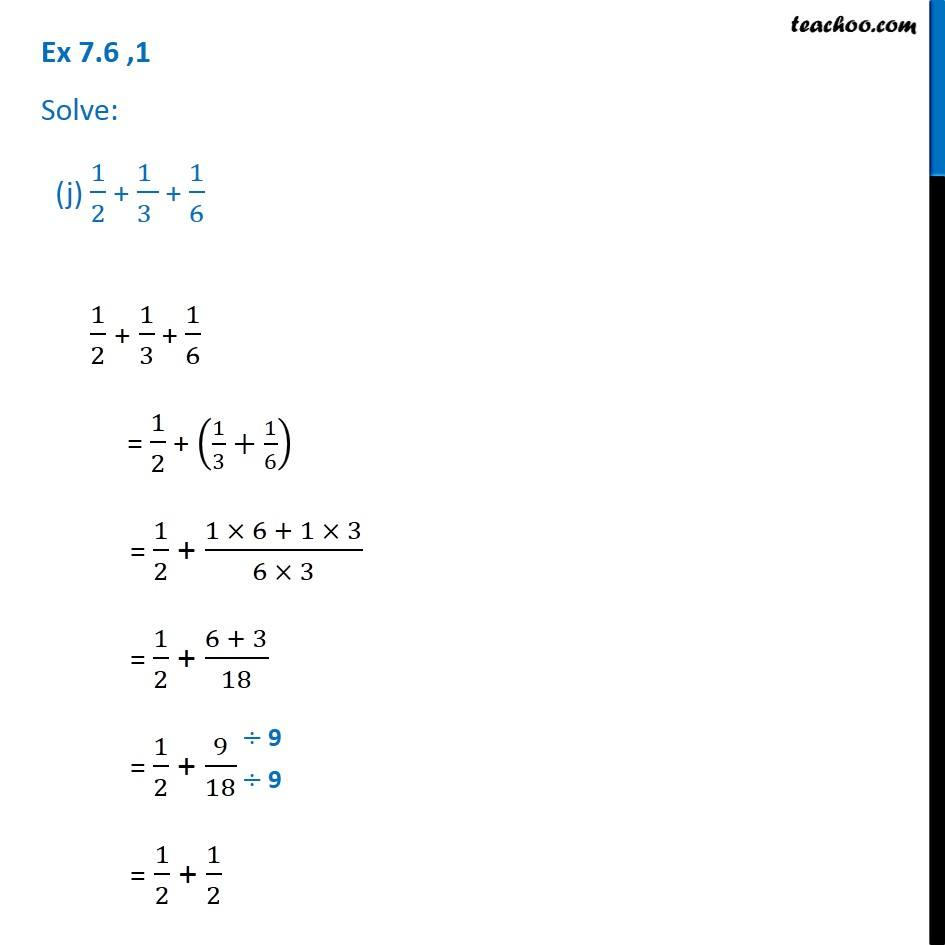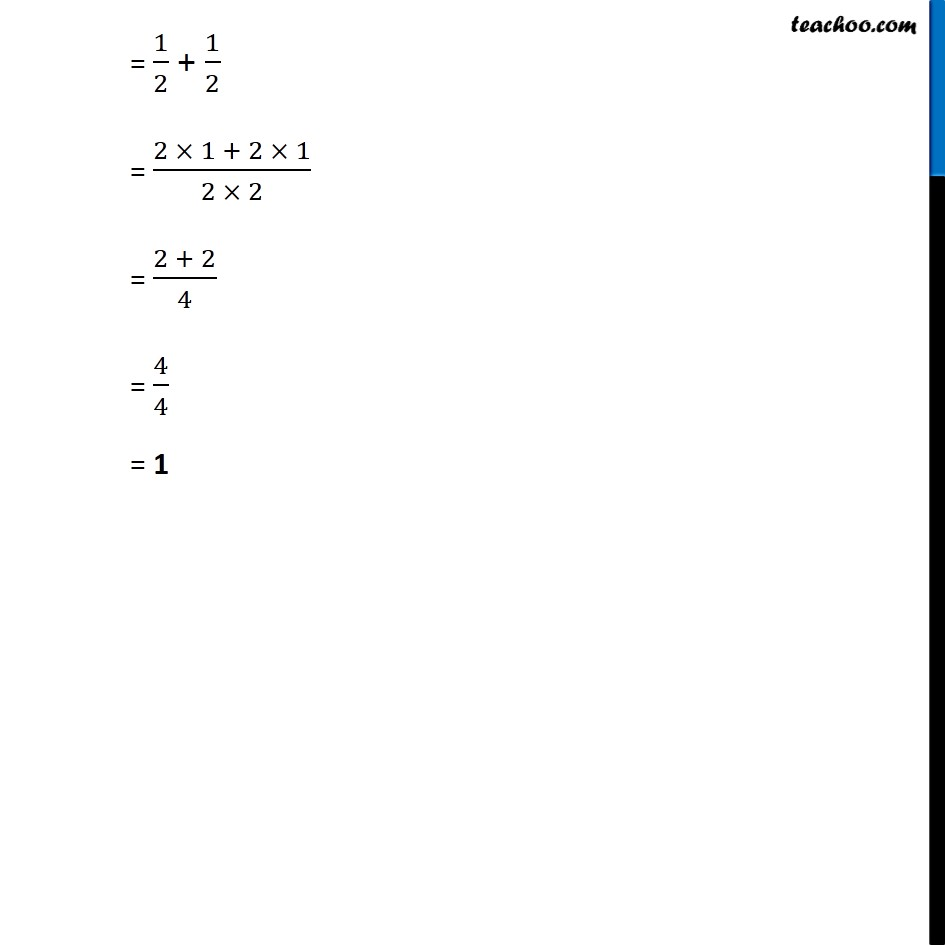1. Chapter 7 Class 6 Fractions
2. Serial order wise
3. Ex 7.6

Transcript

Ex 7.6 ,1 Solve: (j) 1/2 + (1 )/(3 ) + 1/6 1/2 + 1/3 + 1/6 = 1/2 + (1/3+1/6) = 1/2 + (1 6 + 1 3)/(6 3) = 1/2 + (6 + 3)/18 = 1/2 + 9/18 = 1/2 + 1/2 = 1/2 + 1/2 = (2 1 + 2 1)/(2 2) = (2 + 2)/4 = 4/4 = 1